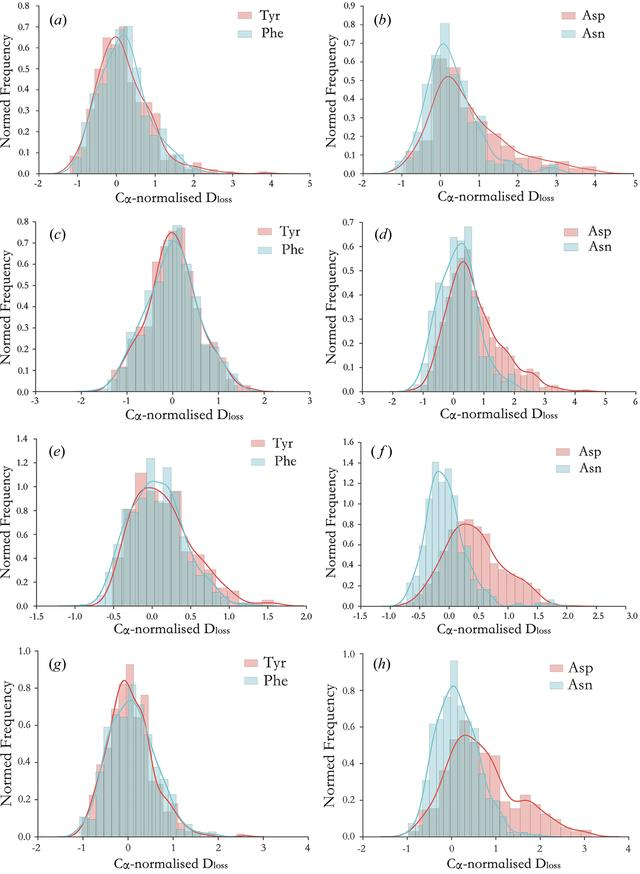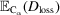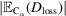disable zoom     view article Figure 4 Cα-normalized Dloss damage signature histogram plots over all atoms of specific residue types for (a, b) myrosinase, (c, d) malate de­hydrogenase, (e, f) TRAP and (g, h) GH7. The normalization scheme is: Cα-normalized Dloss(atom) = [Dloss(atom) −]/, whereis the average Dloss calculated over the set of Cα atoms. Two residue types are directly compared in each plot: (a, c, e, g) Tyr and Phe, and (b, d, f, h) Asp and Asn. Gaussian kernel density estimates, which are non-parametric approximations of the probability density function underlying each Dloss distribution, are overlaid on the histogram plots. Highest dose datasets for each series are displayed; other doses within each series exhibit qualitatively similar behaviour.